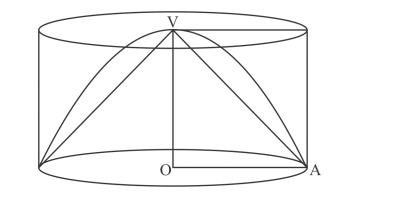# A cone, a hemisphere and a cylinder standQuestion:

A cone, a hemisphere and a cylinder stand on equal bases and have the same height. Show that their volumes are in the ratio 1 : 2 : 3.

Solution:

Let r be the radius of the base.

and h be the height.

Here, h = r.Now,

The ratio of their volumes will be

Volume of cone : volume of hemisphere : volume of a cylinder

$\frac{1}{3} \pi r^{2} h: \frac{2}{3} \pi r^{3}: \pi r^{2} h$

$V_{1}: V_{2}: V_{3}=\frac{1}{3} \pi r^{3}: \frac{2}{3} \pi r^{3}: \pi r^{3}$

Hence, $\quad V_{1}: V_{2}: V_{3}=1: 2: 3$